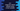# C++ tutorial to swap two numbers without using a third variable# Introduction :

In this C++ example tutorial, we will learn how to swap two numbers without using a third variable. This question is asked in most beginner level interview and you can use the same logic for any programming language.

We can swap two numbers easily by using a third variable. Suppose, the first variable is x and the second variable is y. x is holding 10 and y is holding 20. For swapping these numbers using a third variable z, first of all, set the value of x to this third variable z. Next, set the value of y to x and finally set the value of z to y.

``````Before : x = 10, y = 20
Step 1 : x = 10, y = 20, z = 10
Step 2 : x = 20, y = 20, z = 10
Step 3 : x = 20, y = 10, z = 10``````

It is really easy to do the swapping using a third variable. But how can we do the same thing without using z or the third variable?

## Swap using addition/subtraction or multiplication/division :

Using addition/subtraction (+,-) or using multiplication/division (*,/), we can swap two numbers. Let’s check how to do that :

### Using multiplication/division :

``````Before : x = 10, y = 20
Step 1 : x = x * y
(x = 200, y = 20)

Step 2 : y = x/y
(x = 200, y = 10)

Step 3 : x = x/y
(x = 20, y = 10)
``````

In this example, we have seen how to swap the numbers without using a third number using only multiplication and division. We can also do it using addition and subtraction like below :

``````Before : x = 10, y = 20
Step 1 : x = x + y
(x = 30, y = 20)

Step 2 : y = x - y
(x = 30, y = 10)

Step 3 : x = x - y
(x = 20, y = 10)
``````

Same as the above. Use any of these approaches to swap two numbers.

## C++ program to swap two numbers :

``````#include <iostream>
using namespace std;

int main()
{
int x;
int y;

cout << "Enter the first number :" << endl; cin >> x;
cout << "Enter the second number :" << endl; cin >> y;

cout << "Before swap : x = " << x << ", y = " << y << endl;

//swap
x = x + y;
y = x - y;
x = x - y;

cout << "After swap : x = " << x << ", y = " << y << endl;
return 0;
}
``````

In this example, we are using addition and subtraction to swap the numbers. You can also change it to use multiplication and division.

## Sample Output :

``````Enter the first number :
12
Enter the second number :
25
Before swap : x = 12, y = 25
After swap : x = 25, y = 12
``````

### Conclusion :

This method has more advantage than the other method of using a third number. Using a third number requires more space. So, this process is more space efficient. Try to run the above example and drop one comment below if you have any queries.